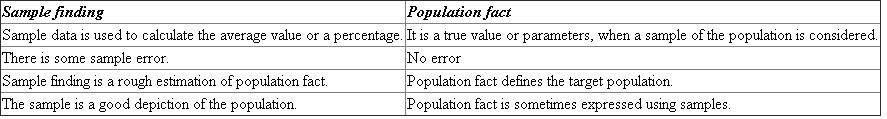# Quiz 12: Generalizing Your Findings

Business

Sample finding helps to calculate the percentage or average value of a sample data. It might include some errors. Population fact shows the exact value of percentage or average calculated for a specific population. Difference between sample finding and population fact is tabulated below:Both the techniques provide a statistical way to determine the quantitative values for a population.

Generalization is the human activity of evaluating a population fact from a sample finding. • It is a set of processes where the sample finding and the sample size are applied to create the evaluations of the population. • It is an outline of logic where an inference on a whole group depends on some validation about that group. Consider the example of a person who migrated to a new city and noted that he is riding faster than other drivers. In this case, generalization depends on watching over the other drivers who were driving slower than this person. Hence, the person would generalize the speed limit followed by other drivers in that city.

Parameter represents the actual value of a population. Parameters are also referred as population fact. It is denoted by a percentage value or an average value. Parameter estimation is the process of estimating the population value. Parameter estimation is also referred as generalization analysis. Information and sample facts are used to estimate the parmeter values.